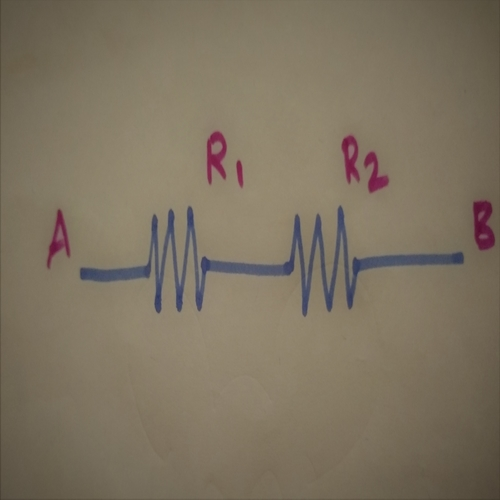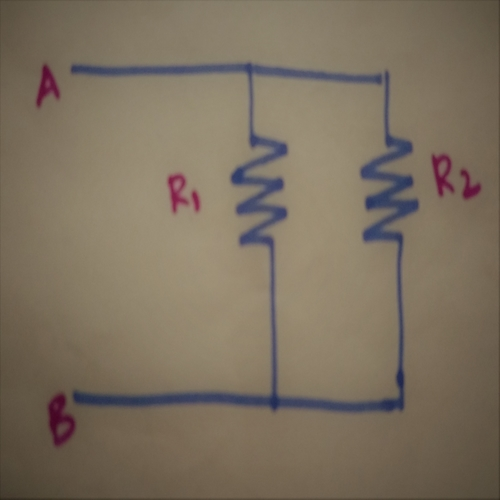# Resistors in Series and Resistors in Parallel Combination

## Resistors in Series:

A combination in which resistors are connected in a sequential manner is called as Resistors in Series. A resistor in series combination possesses the same current throughout the series.This can further be explained by the following expression.

Let  Req be the Equivalent Resistance.

Let R1 and R2 be the Individual Resistances.

V=V1+V2

Req=V/I               (By Ohm’s Law)

Req= (V1+V2)/I

= V1/I  +  V2/I

Req= R1+R2

The Equivalent Resistance of Resistors connected in series is equal to the Sum of Individual Resistances.

## Resistors in Parallel:

A combination in which the Resistors are connected in a parallel (nonsequential manner) is called as Resistors in parallel.  In this parallel combination, the voltage remains same.This can further be explained by the following expression.

Let  Req be the Equivalent Resistance.

Let R1 and R2 be the Individual Resistances.

I=I1 + I2

1/Req  = I/V

1/Req  =I1/V + I2/V

1/Req  = 1/R1 + 1/R2

The reciprocal of Equivalent resistance is equal to the sum of equal to the sum of reciprocals of an individual resistances.

Req      = (R1R2)/(R1+R2)

The Equivalent Resistance of Resistors connected in a parallel combination is equal to the Product of Resistances divided by their sum.

For the sake of explanation, only two resistors are used here. But in practice, there were “n” number of resistors in a circuit. In a circuit, there might be both series and parallel combination.

For “n” number of resistors, it will be difficult to calculate the Equivalent Resistances. So make the calculations user-friendly many theorems were introduced. One can use the above method or theorem depending on the complexity. You will get the same final answer though there would be some approximations on using different methods. For final answers, you can round off the value to the whole number. Theorems will be updated soon.